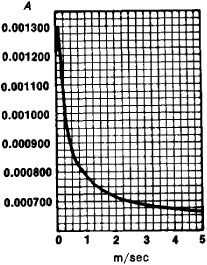# Psychrometric Formula

## psychrometric formula

[¦sī·krə¦me·trik ′fȯr·myə·lə]
(thermodynamics)
The semiempirical relation giving the vapor pressure in terms of the barometer and psychrometer readings.

## Psychrometric Formula

a relationship that permits a determination of vapor pressure from the readings of dry-bulb and wet-bulb thermometers. Mathematically, it is expressed as e = E – AP(ttw), where e is the vapor pressure in the air, E is the saturation vapor pressure at temperature tw ofFigure 1. Dependence of the psychrometer constant A on the velocity of air flow past the thermometer bulb

the wet-bulb thermometer, t is the air temperature, P is the atmospheric pressure, and A is the psychrometer constant, which depends on the design of the psychrometer and, in a more direct way, on the velocity of air flow past the thermometer bulb (see Figure 1).

Mentioned in ?
References in periodicals archive ?
Sanders, 1974: Method for obtaining wet-bulb temperatures by modifying the psychrometric formula. NOAA Tech.
The psychrometric formula relates this observed temperature depression and the temperature of the dry bulb to the quantity of moisture in the air.

Site: Follow: Share:
Open / Close